# Fault Tolerant Control Based SFO-Intelligent PID: Application on SynRM for Flywheel Energy Storage Systems -Part I

Fault Tolerant Control Based SFO-Intelligent PID: Application on SynRM for Flywheel Energy Storage Systems -Part I
EEA Department, National Polytechnic University of Constantine (ENPC), BP 75, A, Nouvelle Ville RP, Constantine, Ain El-Bey road, Constantine 25100, Algeria

Corresponding Author Email:
ilhem.bouchareb@umc.edu.dz
Page:
54-62
|
DOI:
https://doi.org/10.18280/ama_b.641-408
9 July 2021
| |
Accepted:
22 October 2021
| | Citation

OPEN ACCESS

Abstract:

Reliability, safety, and fault-tolerant control (FTC) for power systems are substantially raised in several industrial applications.  It is critical to correctly diagnosis the faults occurring in a drive system to avoid harmful accidents and to ensure continuity of operation. It is necessary to design proper control techniques to recover the faulty system’s performance/functionality to its nominal level. This research work divided into two parts a design scheme of intelligent fault-tolerant control based artificial intelligence techniques (AI) is described and implemented integrates common knowledge acquisition methods with techniques developed in the fields of model-based diagnosis (MBD) to reach the so-called towards intelligent fault tolerant control to provide reliability and maintain the stability of the system in desired performance automatically; This paper presents the first part of this scheme proposes stator-flux oriented (SFO) control with GA-tuned proportional-integral-derivative (PID) controller. The principle is to set the q axis component of stator current invariant during healthy and faulty operating mode. The overall development scheme is summarized and an example illustrates features of the methods performed on a 1.0kW SynRM drive. Results presented have shown that the FT controller scheme developed is potentially capable of dealing with a larger number of faults and can successfully keep a good dynamic performance during faulty mode.

Keywords:

fault tolerant control (FTC), stator-flux oriented control (SFOC), proportional-integral-derivative (PID) control, fault detection, open circuit fault, short circuit fault, power electronics, synchronous reluctance motor (SynRM)

1. Introduction

After 1970s, as the costs of energy increased, the interest of energy accumulators started to grow. Many attempts to use flywheels to store significant quantities of energy were successful in several applications, such as in connection with railways or vehicles or as backup power supplier for devices which should maintain uninterrupted operating in case of power failure [1, 2].

Among the power generators characterized by higher energy and power density, which attracted and still brought more attentions, are the flywheels. The flywheels in recent years, with the help of modern power electronics and high strength materials, high performance flywheels are further developed, different from the earlier times where it were constructed with steel, which had large dimensions and big masses. However, the discussion about the application of this technology is still ongoing concerning their costs, energy density and complexity [3-6].

Flywheels offer virtually unlimited fast charge and fast discharge cycles at high efficiency, and with minimum maintenance requirements, compared with the limited lifetime and lower efficiency of electrochemical batteries. The operating principle of a flywheel energy storage system (FESS) is that electrical energy is converted to kinetic energy and stored in the flywheel, and the kinetic energy can be converted back to electrical energy when required later.

The flywheel rotor design specification is fundamental to the system; if the flywheel inertia is doubled, the energy it stores at a given speed of rotation is doubled, and if the flywheel speed is doubled, the amount of stored energy is quadrupled .

The development of a modular PE drive with adequate control of active and reactive power would allow the exploitation of this FESS concept in grid-connected applications over a range of rated powers .

The well-designed control system may have some fault-tolerance capabilities, it will become more ‘‘active’’ if the fault information can be exploited.  During the past decades, several papers have been published on methods of diagnosing faults, methods of FT control and their applications in various industrial systems and systems, for example [8-34].

In the FT control designs, fault information such as the fault type and magnitude, need to be identified. As some FT controllers heavily relay on real-time fault detection and diagnostics (FDD) schemes to provide the most up-to-date information about the true status of the fault, FDD is especially important. However, most of the research studies on FDD and FC control are carried out as a two separate entities, or the FDD techniques are developed as a monitoring tool, rather than an integral part of the control system. For over- actuated system, not only the fault location, the fault type and magnitude also need to be accurately estimated to achieve a better control performance .

As the fault information, such as the fault type, is usually unknown, it may be limited to design a FT controller for a specified fault. For this reason, it is more desirable to develop a FT controller which can deal with different kinds of actuator faults .

Derivation of the FTC approach requires applications of Ampere's, Ohm's, Faraday’s Laws and the Maxwell Stress Tensor to the multi-path magnetic circuit. The physical requirements of FTC include: decoupling condition, linearity condition, invariance condition where gains are not affected by the failure .

A few FT control methods and limited types of actuator faults are considered for flywheel generator have been previously proposed in the literature [38-46].

One way of achieving fault-tolerance is to employ fault diagnosis schemes on-line. The proposed overall research consists of the following main steps, required to build automatic fault diagnosis system, including (i) fault detection, (ii) fault identification, (iii) fault classification, (iv) fault management and (v) change controller settings to track a desired reference trajectory through maintaining input in the reference model. The block diagram of the system has been shown in Figure 1.

In the first part of research, this paper proposes a control system incorporating stator flux oriented control (SFO) with GA-tuned proportional-integral-derivative (PID) controller to achieve intelligent fault-tolerant control. Once the fault is identified, the supervision scheme will utilize the fault information and pre-computed models to reconfigure the SFO-PID controller. By choosing the ‘‘correct’’ known dynamics; the major changes of system dynamics may be captured faster. By exploiting the information from a fault-diagnosis unit performances of FTC can be further improved.

## 1.png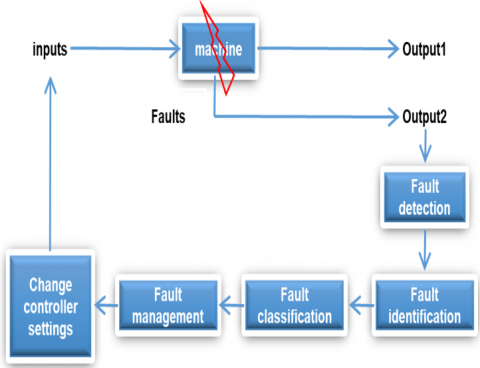Figure 1. Intelligent fault tolerant control technique

2. Flywheel Storage System Description

A flywheel battery acting as an energy buffer is a possible solution for dealing with the fluctuations of wind power. This paragraph describes the selection of different components for a flywheel battery and the design of the motor/generator for the flywheel battery. The components are chosen to reduce cost and increase efficiency. The selected machine technology for the motor/generator in the flywheel battery is the synchronous reluctance machine. A synchronous reluctance motor/generator with low cost, high efficiency, and low rotor weight is designed.  Such conventional flywheels operating at speeds up to 6000 rev/min, together with suitable power converters (drives), electrical motor/generators, and controllers, offer a number of benefits [3-6]:

1. The flywheel rotor can be constructed from low cost steel disks, and may be manufactured and balanced by conventional techniques.
2. The rotor bearings are standard and commercially available, although achieving speeds of 6000 rev/min requires the use of precision high speed bearings.
3. The storage capacity can be increased by adding additional rotor modules (effectively increasing the mass and inertia), giving the potential for high capacity systems.

The major drawback of such systems is that the standing losses, which are mainly due to the use of conventional electrical machines operating in free air, are much higher than electrochemical batteries, making them suitable for short term energy storage only .

The storage system consists of a flywheel, directly connected to a SynR machine, and a power electronic drive, which is connected to the electrical supply. The layout of 1 kW, 48 rpm FESS is shown in Figure 2. The components and electrical machine aimed at flywheel energy storage systems on which FT control was applied are described in detail as following .

## 2.png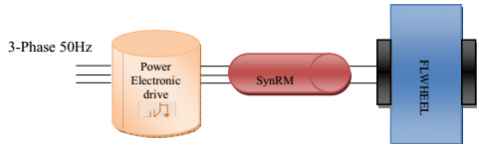Figure 2. Flywheel energy storage system with power electronic drive

The power electronic controller plays a vital role in this system. When the renewable source generates the power, controller sets power flow from renewable to the motor and the motor accelerates the flywheel. The inverter for this setup will have a 200V dc bus, so chosen primarily on safety considerations. The rated current is 60.7 A RMS so as to give a voltage to current ratio similar to the full-scale design. Since the harmonics in the rotor are of particular interest in the small-scale machine the maximum rotational speed and the number of slots will not be changed. Due to these constraints the only way to achieve similar flux densities to the full-scale machine is to make both the rotor to stator air gap and the rotor diameter significantly smaller. The inverter is controlled digitally. The digital controller consists of a combination of a dSPACE® realtime digital signal processor (DSP) controller and a Field Programmable gate Array (FPGA) interface board; Due to this setup hysteretic current control schemes can be easily implemented .

The controller sense the speed of the flywheel and it stops the power flow when flywheel reaches its maximum speed.

An electric machine (E-machine): The stator in the SynR machine is composed of 29 gauge M-15 silicon steel laminations. This steel is chosen for its low iron losses and relatively high saturation flux density. The stator windings are designed as copper bus bars. A standard configuration is preferred where the winding loops slot-to-slot from positive to negative side of the phase and vice-versa. However, fabrication of this in bus bar form is very challenging for the small-scale two pole machine. The machine’s rotor is composed of alternating layers of magnetic steel and nonmagnetic steel. The magnetic layers are made with carbon steel. The grade of carbon steel chosen is AI-4130 due to its electrical resistance (22.3 µ -cm Ω), and good yield strength (161,000 psi) . As shown in Figure 3.

## 3.png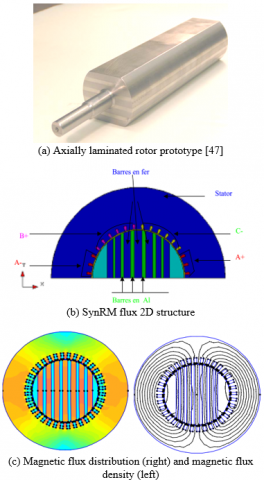Figure 3. SynRM design & FEM characteristics

3. Mathematical Modeling and Analysis

3.1 Mathematical model of a flywheel system

A flywheel stores kinetic energy in a rotating mass. The energy is proportional to the inertia J of the body and in square to the rotating angular speed.

$E=\frac{1}{2} \cdot J \cdot \Omega^{2}$        (1)

The mathematical model of the flywheel system can be described as following:

$J \cdot \frac{d \Omega}{d t}=T_{t}$        (2)

$T_{t}=T_{e}-T_{\text {loss }}$        (3)

$T_{\text {loss }}=f \Omega$        (4)

where, J indicates the rotational inertia of the flywheel, indicates the mechanical speed of the flywheel, Tt indicates the total torque imposed on the flywheel, Te indicates the electrical torque of the SynRM, Tloss indicates the equivalent resistant torque and f indicates the equivalent damping ration.

3.2 Mathematical model of synchronous reluctance machine

In order to develop the equivalent electrical model of the machine, it is necessary to make certain assumptions. These classic assumptions are:

(1)         A sinusoidal distribution of the magnetomotive forces of gap created by the stator and rotor coils.

(2)         Saturation phenomena and magnetic hysteresis are neglected.

(3)         Iron losses in the machine are neglected.

(4)         The skin effect (especially in the rotor bars) is neglected.

(5)         The effect of temperature on the value of the resistors is neglected.

The stator is composed of three coils out of phase by 2p/3 in space. The rotor cage can be modeled by two quadrature windings, one placed along the axis d (low air gap axis) and the other along the axis q. This simplified representation of the rotor does not allow access to the knowledge of the current flowing effectively in each bar but leads to a fairly faithful translation of the influence of the rotor bars on the behavior of the machine. The angle θ represents the mechanical position of the rotor and p the number of pairs of poles.

3.2.1 In fixed stator reference frame

We find a complete development of the model of the unsaturated machine in the stator reference. The expressions of fluxes through the stator and rotor coils are as follows:

$\left[\begin{array}{c} \psi_{s 1} \\ \psi_{s 2} \\ \psi_{s 3} \\ \psi_{r d} \\ \psi_{r q} \end{array}\right]=\left[\begin{array}{ccccc} L_{1}\left(\theta_{e}\right) & L_{12}\left(\theta_{e}\right) & L_{13}\left(\theta_{e}\right) & L_{1 r d}\left(\theta_{e}\right) & L_{1 r q}\left(\theta_{e}\right) \\ L_{21}\left(\theta_{e}\right) & L_{2}\left(\theta_{e}\right) & L_{23}\left(\theta_{e}\right) & L_{2 r d}\left(\theta_{e}\right) & L_{2 r q}\left(\theta_{e}\right) \\ L_{31}\left(\theta_{e}\right) & L_{32}\left(\theta_{e}\right) & L_{3}\left(\theta_{e}\right) & L_{3 r d}\left(\theta_{e}\right) & L_{3 r q}\left(\theta_{e}\right) \\ L_{1 r d}\left(\theta_{e}\right) & L_{1 r d}\left(\theta_{e}\right) & L_{3 r d}\left(\theta_{e}\right) & L_{r d} & 0 \\ L_{1 r q}\left(\theta_{e}\right) & L_{2 r q}\left(\theta_{e}\right) & L_{3 r q}\left(\theta_{e}\right) & 0 & L_{r q} \end{array}\right] \cdot\left[\begin{array}{c} i_{s 1} \\ i_{s 2} \\ i_{s 3} \\ i_{r d} \\ i_{r q} \end{array}\right]$        (5)

The inductances that appear in Eq. (5) depend for the most part on the position of the rotor. As a first approximation, the expressions of the self and mutual inductances of the stator are as follows:

\begin{aligned} &L_{1}\left(\theta_{e}\right)=L_{0}+L_{2} \cos \left(2 \theta_{e}\right) \\ &L_{2}\left(\theta_{e}\right)=L_{0}+L_{2} \cos \left(2\left(\theta_{e}-\frac{2 \pi}{3}\right)\right)' \\ &L_{3}\left(\theta_{e}\right)=L_{0}+L_{2} \cos \left(2\left(\theta_{e}+\frac{2 \pi}{3}\right)\right) \\ &L_{12}\left(\theta_{e}\right)=M_{0}+M_{2} \cos \left(2\left(\theta_{e}+\frac{2 \pi}{3}\right)\right) \\ &L_{23}\left(\theta_{e}\right)=M_{0}+M_{2} \cos \left(2 \theta_{e}\right) \\ &L_{31}\left(\theta_{e}\right)=M_{0}+M_{2} \cos \left(2\left(\theta_{e}-\frac{2 \pi}{3}\right)\right) \end{aligned}        (6)

The expressions of the mutual inductances between the stator windings and the rotor windings are given by:

\begin{aligned} &L_{1 r d}\left(\theta_{e}\right)=M_{s r d} \cos \left(2 \theta_{e}\right) \\ &L_{2 r d}\left(\theta_{e}\right)=M_{s r d} \cos \left(\theta_{e}-\frac{2 \pi}{3}\right)’ \\ &L_{3 r d}\left(\theta_{e}\right)=M_{s r d} \cos \left(\theta_{e}+\frac{2 \pi}{3}\right) \\ &L_{1 r q}\left(\theta_{e}\right)=M_{s r q} \sin \left(\theta_{e}\right) \\ &L_{2 r q}\left(\theta_{e}\right)=M_{s r q} \sin \left(\theta_{e}-\frac{2 \pi}{3}\right) \\ &L_{3 r q}\left(\theta_{e}\right)=M_{s r q} \sin \left(\theta_{e}+\frac{2 \pi}{3}\right) \end{aligned}        (7)

There is a relation between the coefficients L0 and M0 and between the coefficients L2 and M2 appearing in the expression of the own and mutual inductances of the stator:

$\frac{M_{0}}{L_{0}}=-\frac{1}{2}, \frac{M_{2}}{L_{2}}=1$        (8)

The general equations of voltages are obtained by writing the Faraday law for each winding considering the ohmic voltage drop. As the rotor windings equivalent to the cage are short-circuited, the applied voltage is zero:

\begin{aligned} &V_{s a}=R_{s} \cdot i_{a}+\frac{d \psi_{s a}}{d t} \quad 0=R_{r d} \cdot i_{r d}+\frac{d \psi_{r d}}{d t} \\ &V_{s b}=R_{s} \cdot i_{b}+\frac{d \psi_{s b}}{d t}, \quad 0=R_{r q} \cdot i_{r q}+\frac{d \psi_{r q}}{d t} \\ &V_{s c}=R_{s} \cdot i_{c}+\frac{d \psi_{s c}}{d t} \end{aligned}        (9)

The expression of the electromagnetic torque is obtained by derivation of coenergy:

$c e m=\frac{1}{2}[i]^{t} \frac{\partial[L]}{\partial \theta e}[i]$        (10)

This model, valid in the framework of the hypothesis defined previously, can be written more simply in a common reference linked to the rotor.

3.2.2 Model of the machine in the reference (d-q) linked to the rotor

To simplify the equations of the model, one places oneself in a reference linked to the rotor (axes d-q). The stator quantities are reduced to the rotor coordinate system by applying the Park transformation. This mathematical transformation is recalled below for currents. It is perfectly defined whatever θe. It is orthogonal and has an inverse matrix. The normed Park matrix is:

$[\mathrm{P}]=\sqrt{\frac{2}{3}}\left[\begin{array}{ccc} \cos \left(\theta_{e}\right) & \cos \left(\theta_{e}-\frac{2 \pi}{3}\right) & \cos \left(\theta_{e}+\frac{2 \pi}{3}\right) \\ -\sin \left(\theta_{e}\right) & -\sin \left(\theta_{e}-\frac{2 \pi}{3}\right) & -\sin \left(\theta_{e}+\frac{2 \pi}{3}\right) \\ 1 / \sqrt{2} & 1 / \sqrt{2} & 1 / \sqrt{2} \end{array}\right]$        (11)

with

$[\mathrm{P}]^{-1}=[\mathrm{P}]^{t}$        (12)

All the three-phase electrical quantities are projected on the reference linked to the rotor by the Park transformation, we write then in the general case:

$\left[\begin{array}{l} x_{a} \\ x_{b} \\ x_{c} \end{array}\right]=[\mathrm{P}]^{t}\left[\begin{array}{l} x_{d} \\ x_{q} \\ x_{h} \end{array}\right]$        (13)

$\left[\begin{array}{l} i_{S d} \\ i_{S q} \\ i_{S} 0 \end{array}\right]=\sqrt{\frac{2}{3}}\left[\begin{array}{ccc} \cos (\theta e) & \cos \left(\theta e-\frac{2 \pi}{3}\right) & \cos \left(\theta e+\frac{2 \pi}{3}\right) \\ -\sin (\theta e) & -\sin \left(\theta e-\frac{2 \pi}{3}\right) & -\sin \left(\theta e+\frac{2 \pi}{3}\right) \\ 1 / \sqrt{2} & 1 / \sqrt{2} & 1 / \sqrt{2} \end{array}\right]\left[\begin{array}{c} i_{S a} \\ i_{S} b \\ i_{S c} \end{array}\right]$        (14)

Then becomes the system of equations:

$[\mathrm{P}]^{t}\left[v_{d q h}\right]=R_{s}[\mathrm{P}]^{t}\left[\mathrm{I}_{d q h}\right]+[\mathrm{P}]^{t} \frac{d\left[\Psi_{d q h}\right]}{d t}+\frac{d\left[\mathrm{P}^{t}\right]}{d t}\left[\Psi_{d q h}\right]$        (15)

where, [Xdqh] denotes any vector of quantities expressed in the frame of reference tied to the rotor, the indices d, q and h respectively denote the direct components, in quadrature and homopolar. The multiplication of the two members of (15) by [P] gives us:

$[\mathrm{P}]^{t}\left[v_{d q h}\right]=R_{s}[\mathrm{P}]^{t}\left[\mathrm{I}_{d q h}\right]+[\mathrm{P}]^{t} \frac{d\left[\Psi_{d q h}\right]}{d t}+\frac{d\left[\mathrm{P}^{t}\right]}{d t}\left[\Psi_{d q h}\right]$        (16)

with

$[\mathrm{P}] \frac{d[\mathrm{P}]^{t}}{d \theta}=\left[\begin{array}{ccc} 0 & -1 & 0 \\ 1 & 0 & 0 \\ 0 & 0 & 0 \end{array}\right]$        (17)

which give

$\left[v_{d q h}\right]=R_{s}\left[\mathrm{I}_{d q h}\right]+\left[\begin{array}{ccc} L_{d} & 0 & 0 \\ 0 & L_{q} & 0 \\ 0 & 0 & L_{h} \end{array}\right] \frac{d}{d t}\left[\mathrm{I}_{d q h}\right]+p \Omega\left[\begin{array}{ccc} 0 & -L_{q} & 0 \\ L_{d} & 0 & 0 \\ 0 & 0 & 0 \end{array}\right]\left[\mathrm{I}_{d q h}\right]$        (18)

or,

$\left[\begin{array}{ccc} L_{d} & 0 & 0 \\ 0 & L_{q} & 0 \\ 0 & 0 & L_{h} \end{array}\right]=[\mathrm{P}][L][\mathrm{P}]^{t}$        (19)

with

$\left\{\begin{array}{l} L_{d}=L_{0}+L_{f}-M_{0}+\frac{1}{2} L_{2}+M_{2} \\ L_{q}=L_{0}+L_{f}-M_{0}-\frac{1}{2} L_{2}+M_{2} \\ L_{h}=L_{0}-M_{0} \end{array}\right.$        (20)

Since the neutral of the machine is isolated, which naturally implies ih = 0, we can write:

$\left[\begin{array}{l} v_{d} \\ v_{q} \end{array}\right]=\left[\begin{array}{cc} R_{s} & -p \Omega L_{q} \\ p \Omega L_{d} & R_{s} \end{array}\right]\left[\begin{array}{c} i_{d} \\ i_{q} \end{array}\right]+\left[\begin{array}{cc} L_{d} & 0 \\ 0 & L_{q} \end{array}\right] \frac{d}{d t}\left[\begin{array}{c} i_{d} \\ i_{q} \end{array}\right]$        (21)

$\frac{d}{d t}\left[\begin{array}{c} i_{d} \\ i_{q} \end{array}\right]=\left[\begin{array}{cc} \frac{-R_{s}}{L_{d}} & \frac{p \Omega L_{q}}{L_{d}} \\ -\frac{p \Omega L_{d}}{L_{q}} & \frac{-R_{s}}{L_{q}} \end{array}\right]\left[\begin{array}{c} i_{d} \\ i_{q} \end{array}\right]+\left[\begin{array}{cc} \frac{1}{L_{d}} & 0 \\ 0 & \frac{1}{L_{q}} \end{array}\right]\left[\begin{array}{l} v_{d} \\ v_{q} \end{array}\right]$        (22)

3.2.3 Mechanical equations

The electromagnetic couple is derived, according to the principle of virtual works, from the expression of coenergy and in a linear mode of operation:

$\mathrm{Cem}=\frac{1}{2} p[I a b c]^{t}\left[\frac{\partial L}{\partial \theta}\right][I a b c]$        (23)

The replacement of the current vector by its Park transform gives us:

$\left\{\begin{array}{l} \mathrm{Cem}=\frac{1}{2}[I d q h]^{t}[\mathrm{P}]^{t}\left[\frac{\partial L}{\partial \theta}\right]\left[I_{d q h}\right] \\ =\frac{1}{2} p[I d q h]^{t}\left[\begin{array}{ccc} 0 & -L_{q} & 0 \\ L_{d} & 0 & 0 \\ 0 & 0 & 0 \end{array}\right]\left[I_{d q h}\right] \\ =p\left(L_{d}-L_{q}\right) i_{d} i_{q} \end{array}\right.$        (24)

The equation of electromagnetic torque is associated with the fundamental relationship of the dynamics of rotating systems:

$J \frac{d \Omega}{d t}+f \Omega=C e m-C r$        (25)

where, W: the rotational speed of the rotor, J: the moment of inertia of the system, f: the coefficient of viscous friction, Cem: the electromagnetic couple, Cr: the resistant torque.

The equivalent electrical model of the machine in the common reference of the rotor is relatively a simple model, obtained where the machine is represented by two coupled electrical circuits, one along the axis d and another along the axis q. In the following, we will not consider the equations relating to the homopolar component (machine coupled in star without neutral). The equations of the stator voltages at the terminals of the equivalent windings of axis d and axis q are written:

\begin{aligned} &U_{s d}=R_{s} \cdot i_{s d}+\frac{d \psi_{s d}}{d t}-\omega_{e . \psi_{s q}} \\ &U_{s q}=R_{s} \cdot i_{s q}+\frac{d \psi_{s q}}{d t}+\omega_{e . \psi_{s d}} \end{aligned}        (26)

The flows used in the stator windings are connected to the currents by the following relations:

\begin{aligned} &\psi s d=L d . i s d+\text { Md.ird } \\ &\psi s q=L q . i r q+\text { Mq.irq } \end{aligned}        (27)

In the same way, one can write the equations of the rotor voltages (the equivalent windings are in short-circuit):

\begin{aligned} &0=R r d . i r d+\frac{d \psi r d}{d t} \\ &0=R r q \cdot i r q+\frac{d \psi r q}{d t} \end{aligned}        (28)

The flows used in the rotor windings are written:

\begin{aligned} &\psi r d=\text { Lrd.ird }+\text { Md.isd } \\ &\psi r q=\text { Lrq.irq }+\text { Mq.isq } \end{aligned}        (29)

It is shown that the expression of the electromagnetic torque developed by the machine can be written in the following way:

$\text { cem }=\mathrm{P}.(\psi s d . i s q-\psi s q . i s d)$        (30)

The resulting electromechanical model MSRV in the form of state equations:

$\frac{d}{d t}\left[\begin{array}{c} i d \\ i q \\ \Omega \\ \theta \end{array}\right]=\left[\begin{array}{c} \frac{-R s}{L d} i d+\frac{p L q}{L d} i q \Omega \\ \frac{-R s}{L q} i q-\frac{p L d}{L q} i d \Omega \\ \frac{p(L d-L q) i d i q}{J}-\frac{f}{J} \Omega-\operatorname{Tr} \\ p \Omega \end{array}\right]+\left[\begin{array}{cc} \frac{1}{L d} & 0 \\ 0 & \frac{1}{L q} \\ 0 & 0 \\ 0 & 0 \end{array}\right]\left[\begin{array}{l} v d \\ v q \end{array}\right]$        (31)

4. Fault Tolerant Control Strategy

The main objective of this part is to propose fault-tolerant control architectures of a wind turbine conversion chain with a variable reluctance synchronous machine (SynRM), in the presence of several faults.

## 4.png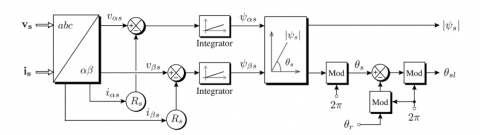Figure 4. Principle of stator-flux oriented vector control

The fault tolerant control (FTC) ensures an acceptable operating mode even in the presence of faults. This fault tolerance can be provided by conventional robust control techniques. We propose to implement a SFT Stator-Flux-Oriented Fault-Tolerant Control strategy for fault-tolerant operation of SynRMs as it was explained and applied for stator-flux-oriented fault-tolerant control of flux-switching permanent-magnet motors in the research paper  with the following equations from (32) til (37). The main idea is to put the armature currents on the invariant axis q before and after the appearance of the defect. Oriented flux control as presented in Figure 4 is a technique which, despite the complex and non-linear structure of the machine, allows its control in a similar way to that of the DC machine. Therefore, three types of machine oriented flow control are possible: Orientation of the rotor flow; Orientation of the stator flux and orientation of the flux of gap. Each of these control methods provides decoupled flow control and torque control such as a separate excitation DC machine. This decoupling makes it possible to obtain very good responses of the drive in dynamic mode.

In the context of this work, we develop the vector control with reference orientation (d-q) following the stator flux. This type of control is used "The stator-oriented flow control" to obtain the desired operating mode by optimally positioning the current vectors.

Based on the proposed SFO-dq control, SynR motor currents in normal operation can be expressed as follows:

$\left\{\begin{array}{l} i_{a}=i_{d} \cos \theta-i_{q} \sin \theta \\ i_{b}=i_{d} \cos \left(\theta-\frac{2 \pi}{3}\right)-i_{q} \sin \left(\theta-\frac{2 \pi}{3}\right) \\ i_{c}=i_{d} \cos \left(\theta+\frac{2 \pi}{3}\right)-i_{q} \sin \left(\theta+\frac{2 \pi}{3}\right) \end{array}\right.$         (32)

The transformation αβ0/abc can be written as well:

$\left[\begin{array}{l} i_{a} \\ i_{b} \\ i_{c} \end{array}\right]=\left[\begin{array}{ccc} 1 & 0 & 1 \\ -\frac{1}{2} & \frac{\sqrt{3}}{2} & 1 \\ -\frac{1}{2} & -\frac{\sqrt{3}}{2} & 1 \end{array}\right] \cdot\left[\begin{array}{l} i_{\alpha} \\ i_{\beta} \\ i_{0} \end{array}\right]$         (33)

When an open circuit fault occurs in the phase 'a' the current ‘ia’ is vanished so that the motor is powered by the two healthy phases. The homopolar component of the armature current can be obtained:

$i_{0}=-i_{\alpha}$         (34)

Then, substituting (34) in (33), deduces

$\left\{\begin{array}{l} i_{a}^{\prime}=0 \\ i_{b}^{\prime}=-\frac{3}{2} i_{\alpha}+\frac{\sqrt{3}}{2} i_{\beta} \\ i_{c}^{\prime}=-\frac{3}{2} i_{\alpha}-\frac{\sqrt{3}}{2} i_{\beta} \end{array}\right.$         (35)

The transformation dq/αβ can be written as well:

$\left[\begin{array}{l} i_{\alpha} \\ i_{\beta} \end{array}\right]=\left[\begin{array}{cc} \cos \theta & -\sin \theta \\ \sin \theta & \cos \theta \end{array}\right] \cdot\left[\begin{array}{l} i_{d} \\ i_{q} \end{array}\right]$         (36)

Then, substituting (36) in (35), the strategy of the tolerant command can be deduced:

$\left\{\begin{array}{l} i_{a}^{\prime}=0 \\ \dot{\boldsymbol{i}}_{b}^{\prime}=\sqrt{3}\left[i_{d} \cos \left(\theta-\frac{5 \pi}{6}\right)-i_{q} \sin \left(\theta-\frac{5 \pi}{6}\right)\right] \\ \dot{l}_{c}^{\prime}=\sqrt{3}\left[i_{d} \cos \left(\theta+\frac{5 \pi}{6}\right)-i_{q} \sin \left(\theta+\frac{5 \pi}{6}\right)\right] \end{array}\right.$         (37)

Performance in normal and fault-tolerant operation is tested, confirming that the proposed fault-tolerant control can maintain the invariant torque while providing good dynamic performance in the event of a fault.

During normal and fault-tolerant operation of the SynR machine, the co-simulated torque and current waveforms are obtained as shown in the Figure 8. We see that in normal operation, the average torque (Tav) and Torque ripple (KT) of the engine are 5.0142 Nm and 50%, respectively. From these results it can be concluded that the normal and fault-tolerant torque performance is virtually unchanged.

$K_{T}=\frac{T_{\max }-T_{\min }}{T_{a v}} \times 100 \%$         (38)

One of the main limitations of an SFOC structure is its relatively weaker fault accommodation capability. In order to overcome this shortcoming and in order to obtain a robust FTC structure, SFOC combined with a intelligent PID controller tuned by a GA is introduced in the feedforward loop of the SFOC scheme by exploiting the power of PID for tracking control of SynRM rotor speed Figure 6.

When a fault occurs (SC or OC), the system dynamics will change, and in order to maintin the system to its healthy state and it perfomance means the continuity of producing torque we integerate genetic algorithms work by setting the error value to re-correct parameters of PID and the post-fault system will follow the behavior specified to maintain the same or as close as possible performance when there are no faults. This in fact is the principal rationale of using PID tuned by a GA for FTC of the SynRM, and for FTC systems of Flywheel Energy Storage Systems in general (Figure 7).

As can be observed in Figure 8, the proposed FTC scheme in Figure 5 can still maintain the desired torque after fault occurrence (open circuit in phase a), while the baseline controller fails to maintain the torque and ﬁnally makes the system become unstable. In this structure, the inteligent PID controller helps to leads a biased steady-state error and obtain the desired settling time and rise time.

The uses of SFOC-intelligent PID controllers offer the advantage of accommodating any deviation of the system from the reference response, either these are faults or perturbations. Therefore, an FDD scheme is not necessary for such an FTC system, making the FTC system simpler and heavily relies on reference model design.

## 5.png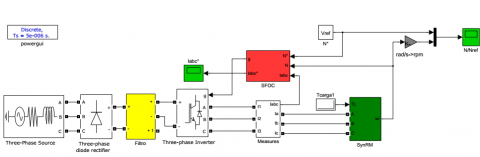Figure 5. Control block diagram of fault-tolerant SynR motor drive

## 6.png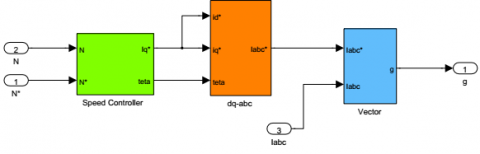Figure 6. Sub block control diagram of fault-tolerant SynR motor drive

## 7.png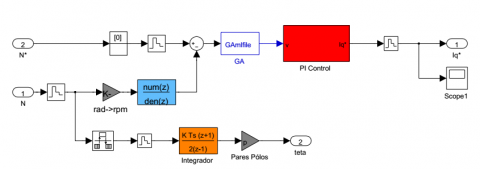Figure 7. Sub block control diagram of PID controller tuned by a GA

## 8.png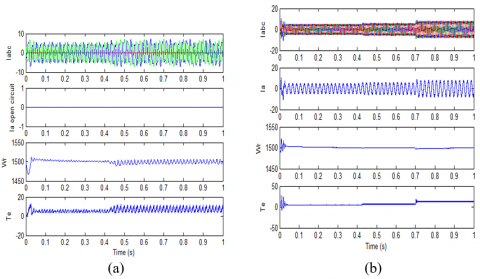Figure 8. Simulation in healthy operation: currents, speed and torque (a) fault tolerant operation (b)

5. Conclusions

The main contribution of this paper is to implement of an intelligent FT controller on SynRM aimed at flywheel energy storage systems, rotating at speeds up to 6000 rev/min, and primarily targeted at renewable energy applications such as wind and wave.

The complete FESS simulation model is the integration of different subsystems essential for the analysis as discussed.

It was veriﬁed by extensive simulations with a variety of operational conditions that the output torque maintain constant and stability of dynamic performance is not lost for a wide range of additive faults, although the performance characteristics may diﬀer.

The obtained results show the efficiency of the proposed control approach for the detection, diagnosis, and the classification of faults in SynRM ….

Through this research for more complicated problem FCO based fault tolerant control simulations indicate that the approach is applicable to a class of multiplicative faults and the properties of the control loop in this context will be analyzed in a future work.

This approach can be applied without taking into account the type of machine or type of fault. Finally, torque ripple analysis proves the effectiveness of this method.

References

 Genta, G. (1985). Kinetic Energy Storage: Theory and Practice of Advanced Flywheel Systems. 1st. ed. London, UK & Boston, USA: Butterworths.

 Li, X. (2019). Evaluation and Design of a Flywheel Energy Storage System. Phd Dissertation.

 Erd, N., Li, X., Binder, A. (2017). Power flow simulation of flywheel energy storage systems for tramways. Renewable Energy and Power Quality Journal, 1(15): 149-153. http://www.icrepq.com/icrepq17/256-17-erd.pdf.

 Li, X., Erd, N., Binder, A., (2017). Design and calculation of a 130 kW high-speed permanent magnet synchronous machine in flywheel energy storage systems for urban railway application. In Proc. 6th International Conference on Clean Electrical Power (ICCEP), Santa Margherita Ligure, Italy, pp. 452-459. https://doi.org/10.1109/ICCEP.2017.8004727

 Li, X., Erd, N., Binder, A. (2016). Evaluation of flywheel energy storage systems for residential photovoltaic installations. in Proc. International Symposium on Power Electronics, Electrical Drives, Automation and Motion (SPEEDAM), Anacapri, Italy, pp. 255-260. https://doi.org/10.1109/SPEEDAM.2016.7525914

 Li, X., Erd, N., An, J., Messager, G., Binder, A. (2017). Design of a high-speed flywheel energy storage demonstrator. in Proc. 7th VDE/VDI Symposium on Drive Systems, Karlsruhe, Germany, pp. 19-24.

 Ruddell, A.J., Schoennenbeck, G.S., Bleijs, J.A.M. (1998). Power converters for flywheel energy storage systems. JOULE PROJECT JOR3-CT95-0070. https://cordis.europa.eu/project/id/JOR3950070

 Vas, P. (1993). Parameter Estimation, Condition Monitoring and Diagnosis of Electrical Machines. Oxford, U.K.: Clarendon.

 Thompson, W.T. (1999). Review of on line condition monitoring techniques for three squirrel induction motors-past, present and future. Proc. of the IEEE SDEMPED international symposium. Diagnostics for Electric Machines, Power Electronics and Drives, pp. 7-9.

 Carrasco, J.A. (2006). Failure transition distance-based importance sampling schemes for the simulation of repairable fault-tolerant computer systems. IEEE Transactions on Reliability, 55(2): 207-236. https://doi.org/10.1109/TR.2006.874910

 Ahmed-Zaid, F., Ioannou, P., Gousman, K., Rooney, R. (1991). Accommodation of failures in the F-16 aircraft using adaptive control. IEEE Control Systems Magazine, 11(1): 73-78. https://doi.org/10.1109/37.103360

 Hamada, Y., Shin, S., Sebe, N. (1996). A design method for fault-tolerant control systems based on H∞ optimization. Proc. of the 35th Conference on Decision and Control (CDC’96), Kobe, Japan, pp. 1918-1919. https://doi.org/10.1109/CDC.1996.572857

 Isermann, R., Balle, P. (1997). Trends in the application of model-based fault detection and diagnosis of technical processes. Control Engineering Practice, 5(5): 709-719.

 Balle, P., Fischer, M., Fussel, D., Nelles, O., Isermann, R. (1998). Integrated control, diagnosis and reconfiguration of a heat exchanger. IEEE Control Systems Magazine, 18(3): 52-63. https://doi.org/10.1109/37.687620

 Chen, J., Patton, R. (1999). Robust Model-Based Fault Diagnosis for Dynamic Systems. Kluwer. book, Springer.

 Maron, D., Hamelin, V., Noura, F., (1999). A fault-tolerant control design against major actuator failures: Application to a three tank system. Proc. of the 38th IEEE Conference on Decision and Control (CDC’99), pp. 3569-3574. https://doi.org/10.1109/CDC.1999.827906

 Zamanabadi, R., Staroswiecki, M., (2000). A structural analysis method formulation for fault-tolerant control system design. Proc. of the 39th IEEE Conference on Decision and Control (CDC’00), pp. 4901-4902. https://doi.org/10.1109/CDC.2001.914707

 Bolognani, S., Zordan, M., Zigliotto, M. (2000). Experimental fault-tolerant control of a PMSM drive. IEEE Transactions on Industrial Electronics, 47(5): 1134-1141. https://doi.org/10.1109/41.873223

 Diao, Y., Passino, K., (2001). Fault tolerant stable adaptive fuzzy/neural control for a turbine engine. International Journal of IEEE Transactions on Control Systems Technology 9(3): 494–509. https://doi.org/10.1109/87.918902

 Ho, L.W., Yen, G.G. (2002). Reconfigurable control system design for fault diagnosis and accommodation. International Journal of Neural Systems, 12(06): 497-520. https://doi.org/10.1142/s0129065702001333

 Ferreira, P. (2002). Tracking with sensor failures. International Journal of Automatica, 38(9): 1621-1623. https://doi.org/10.1016/S0005-1098(02)00070-5

 Diao, Y., Passino, K.M. (2002). Intelligent fault-tolerant control using adaptive and learning methods. Control Engineering Practice, 10(8): 801-817. https://doi.org/10.1016/S0967-0661(02)00032-1

 Blanke, M., Kinnaert, M., Lunze, J., Staroswiecki, M. (2003). Diagnosis and fault-tolerant control. International Journal of Springer, Book. https://doi.org/10.1007/978-3-540-35653-0

 Benbouzid, M.E.H., Diallo, D., Zeraoulia, M. (2007). Advanced fault-tolerant control of induction-motor drives for EV/HEV traction applications: From conventional to modern and intelligent control techniques. IEEE Transactions on Vehicular Technology, 56(2): 519-528. https://doi.org/10.1109/TVT.2006.889579

 Garza-Castañón, L.E., Vargas-Martínez, A. (2010). Artificial intelligence methods in fault tolerant control. Chapter of Book Automation and Control-Theory and Practice, Ed. In-TECH, Austria.

 Liu, M., Cao, X., Shi, P. (2012). Fuzzy-model-based fault-tolerant design for nonlinear stochastic systems against simultaneous sensor and actuator faults. IEEE Transactions on Fuzzy Systems, 21(5): 789-799. https://doi.org/10.1109/TFUZZ.2012.2224872

 Hadroug, N., Hafaifa, A., Batel, N., Kouzou, A., Chaibet, A. (2018). Active fault tolerant control based on a neuro fuzzy inference system applied to a two shafts gas turbine. Applied Artificial Intelligence, 32(6): 515-540. https://doi.org/10.1080/08839514.2018.1483114

 Alves Jr, M.A.O., Nobrega, E.G., Yoneyama, T. (2009). Adaptive neural control for a tolerant fault system. IFAC Proceedings Volumes, 42(8): 137-142. https://doi.org/10.3182/20090630-4-ES-2003.00023

 El-Koujok, M., Gouriveau, R., Zerhouni, N. (2009). Error estimation of a neuro-fuzzy predictor for prognostic purpose. IFAC Proceedings Volumes, 42(8): 131-136. https://doi.org/10.3182/20090630-4-ES-2003.00022

 Witczak, M., Pazera, M. (2016). Fault tolerant-control: Solutions and challenges. Pomiary Automatyka Robotyka, 20(1): 5-16. http://dx.doi.org/10.14313%2FPAR_219%2F5

 Naik, A.S., Yin, S., Ding, S.X., Jeinsch, T. (2009). Recursive identification algorithm for parity space based fault detection systems. IFAC Proceedings Volumes, 42(8): 312-317. https://doi.org/10.3182/20090630-4-ES-2003.00052

 Odgaard, P.F., Stoustrup, J., Kinnaert, M. (2013). Fault-tolerant control of wind turbines: A benchmark model. IEEE Transactions on Control Systems Technology, 21(4): 1168-1182. https://doi.org/10.1109/TCST.2013.2259235

 Yu, R., Breikin, T., Wang, H. (2009). A hybrid ANN-Based fault diagnosis and tolerance method and its application. IFAC Proceedings Volumes, 42(8): 378-383. https://doi.org/10.3182/20090630-4-ES-2003.00063

 Jain, T. (2012). Behavioral System-theoretic approach to fault-tolerant control (Doctoral dissertation, Université de Lorraine).

 Verhaegen, M., Kanev, S., Hallouzi, R., Jones, C., Maciejowski, J., Smail, H. (2010). Fault tolerant flight control-a survey. In Fault Tolerant Flight Control, pp. 47-89. https://doi.org/10.1007/978-3-642-11690-2_2

 Li, M.H., Palazzolo, A.B., Kenny, A., Provenza, A.J., Beach, R.F., Kascak, A.F. (2004). Fault-tolerant homopolar magnetic bearings. IEEE Transactions on Magnetics, 40(5): 3308-3318. https://doi.org/10.1109/TMAG.2004.833428

 Abdin, E.S., Xu, W. (2000). Control design and dynamic performance analysis of a wind turbine-induction generator unit. IEEE Transactions on Energy Conversion, 15(1): 91-96. https://doi.org/10.1109/60.849122

 Samineni, S., Johnson, B.K., Hess, H.L., Law, J.D. (2006). Modeling and analysis of a flywheel energy storage system for voltage sag correction. IEEE Transactions on Industry Applications, 42(1): 42-52. https://doi.org/10.1109/TIA.2005.861366

 De Andrade, R., Sotelo, G.G., Ferreira, A.C., et al. (2007). Flywheel energy storage system description and tests. IEEE Transactions on Applied Superconductivity, 17(2): 2154-2157. https://doi.org/10.1109/TASC.2007.899056

 Arai, J., Iba, K., Funabashi, T., Nakanishi, Y., Koyanagi, K., Yokoyama, R. (2008). Power electronics and its applications to renewable energy in Japan. IEEE Circuits and Systems Magazine, 8(3): 52-66. https://doi.org/10.1109/MCAS.2008.928420

 Vargas-Martínez, A., Avila, L.I.M., Zhang, Y., Garza-Castañón, L.E., Ortiz, E.R.C. (2013). Model-based fault-tolerant control to guarantee the performance of a hybrid wind-diesel power system in a microgrid configuration. Procedia Computer Science, 19: 712-719. https://doi.org/10.1016/j.procs.2013.06.094

 Yu, J., Zhu, C. (2016). A Sensor-fault tolerant control method of active magnetic bearing in flywheel energy storage system. In 2016 IEEE Vehicle Power and Propulsion Conference (VPPC), Hangzhou, China, pp. 1-6. https://doi.org/10.1109/VPPC.2016.7791595

 Kamal, E., Aitouche, A., Oueidat, M. (2013). Fuzzy fault-tolerant control of wind-diesel hybrid systems subject to sensor faults. IEEE Transactions on Sustainable Energy, 4(4): 857-866. https://doi.org/10.1109/TSTE.2013.2253138

 Daoud, M.I., Abdel-Khalik, A.S., Elserougi, A., Massoud, A., Ahmed, S. (2014). Medium voltage flywheel energy storage system employing dual three-phase induction machine with machine-side series-connected converters. Proc. of the 7th IET International Conference on Power Electronics, Machines and Drives (PEMD 2014). https://doi.org/10.1049/cp.2014.0394

 Romero, M., Seron, M.M., De Doná, J.A. (2009). Sensor fault tolerant direct torque and flux control of induction motors. IFAC Proceedings Volumes, 42(8): 173-178. https://doi.org/10.3182/20090630-4-ES-2003.00029

 Mohan, N. (2004). Enhancing the dispatchability of wind energy using inertial storage and hybrid systems. Report Department of Electrical Engineering University of Minnesota.

 Zhao, W.X., Cheng, M., Chau, K.T., Hua, W., Jia, H.Y., Ji, J.H., Li, W.L. (2011). Stator-flux-oriented fault-tolerant control of flux-switching permanent-magnet motors. IEEE Transactions on Magnetics, 47(10): 4191-4194. https://doi.org/10.1109/TMAG.2011.2157106